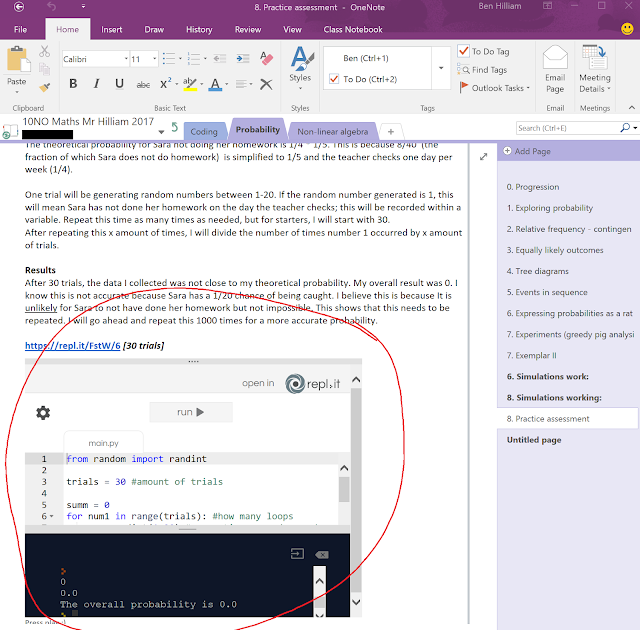# Guest Post: Surprises when using Office 365 in class

Today's post comes from Mr Ben Hilliam, Head of Junior Maths at St Andrew's College in Christchurch, New Zealand. In this post he outlines how he is teaching basic programming concepts using Microsoft Excel and also the increasing versatility of Microsoft OneNote through the use of embedded applets that can show and execute Python coding.

You can read the original post from Ben here - please do check out his other posts as well. I particularly like Ben's finishing comment when it comes to describing OneNote:

OneNote is more than a piece of digital paper, it is quickly becoming the universal canvas of expression for learning.

Here's the entirety of the post:

With a new year I have begun teaching some new topics. For the first time in my career, I am teaching Probability Simulations to year 10s. As usual, OneNote is my go to, where I drop all my resources so that my students can access the problems I set them. In this topic I have come across two surprises:

1. Microsoft Excel is still a very cool program
2. Microsoft OneNote allows for a huge range of student expression

1. Microsoft Excel is still a very cool program:

One task I set my year 10s was the following:

Sara has maths on four days each week.
Her teacher checks the class's homework on only one of those days.
The day is chosen at random by the teacher.
Students who have not done their homework for that day get a detention.
Sara says she did her homework on 32 out of the 40 days on which she had maths last term.
Describe a simulation (probability experiment) that Sara could use to predict the probability
that:
(1) she had not done her homework
AND
(2) the teacher checked.
Assume that you have access to: coins, cards, spinners, dice and a random number
generator on a calculator or computer.
You must give sufficient detail in your description so that someone else could carry out
the experiment.
You must state what you will record and how you will calculate the probability.

Most of my students used Excel to run this simulation. One problem that quickly made itself apparent was how do you get the teachers to randomly check on one day of the week only, becuase most students assigned random numbers of 1-4 on each day which meant the teacher could potentially check homework more than once in any given week.

This is where Excel comes to the fore, with its conditional formulae:

A "1" comes up when an even that we are interested in occurs while a "0" comes up for events that are irrelevant. Where two events occur at the same time they add to 2 which is then picked up. Here's the formula that makes it work:The point I'm trying to make here is that if you have a problem that requires programming, Excel can actually do it. With a few choice "if" statements and other formulae, my students were able to produce a simulation that correctly estimated the probability they were investigating. So they could be accurate in their thinking rather than smudge a "near enough" response.So don't forget Excel, it's awesome.

2. Microsoft OneNote allows for a huge range of student expression

Last year, I did some coding with my year 9s. Specifically we had a look at solving problems from Project Euler using Python. So when we started doing Simulations, some students naturally wanted to use Python to run their simulations. My question to them was "How will you show me the results of your simulation?" Their response: embed an HTML window in OneNote with their Python code running in an applet:In the part I have circled in red, my student has imported an HTML window with her Python code and an applet that will run it. This meant I was able to see the output of their simulation and check it was consistent with what was expected and I could look through their code if there was a problem. Such a simple addition to OneNote has such wide consequences for how we use it.

OneNote is more than a piece of digital paper, it is quickly becoming the universal canvas of expression for learning.